# Fractal Circles

August 2014 // Re:GENERATE

In August of 2014, I participated in Re:GENERATE, a show featuring computer-generated art. I made, among other things, a generative series for the event, that I named Fractal Circles.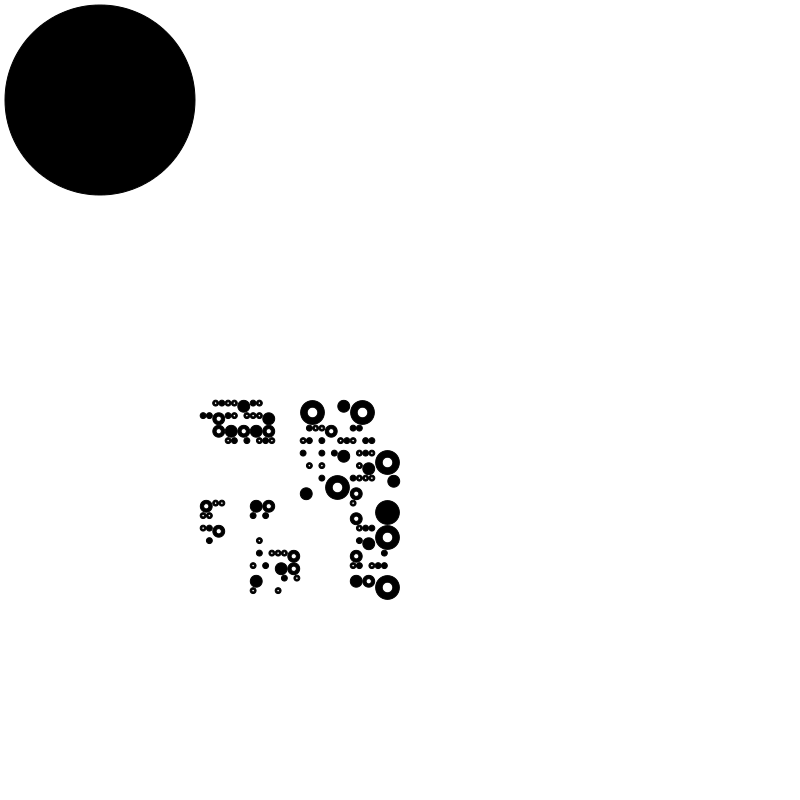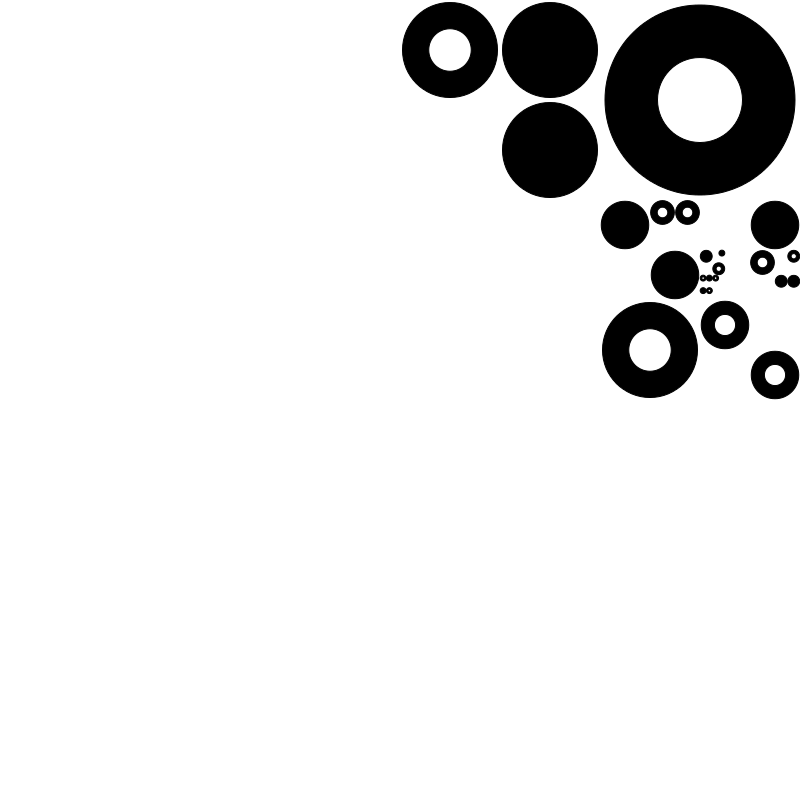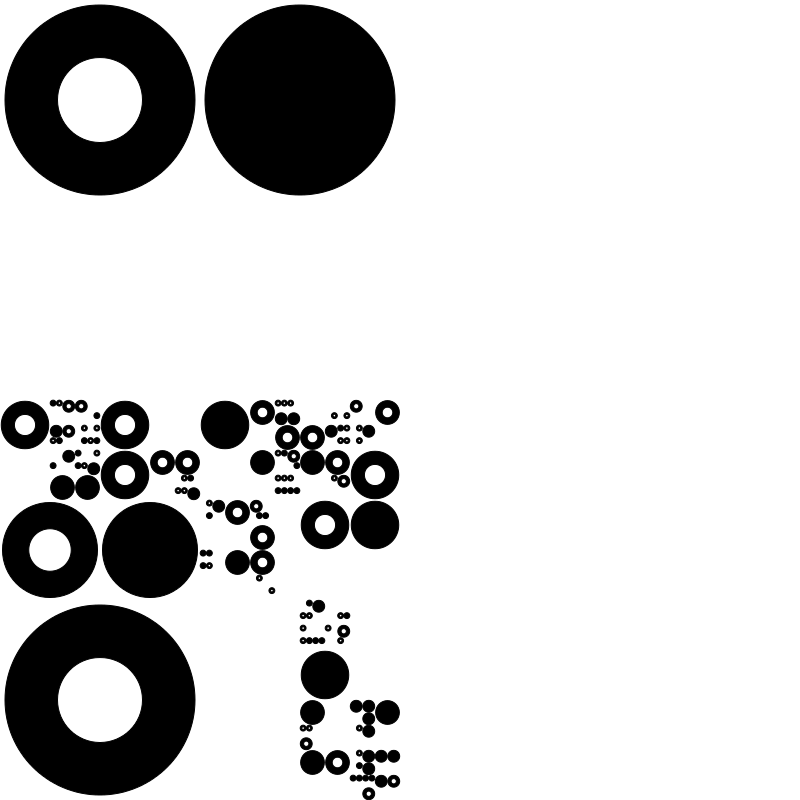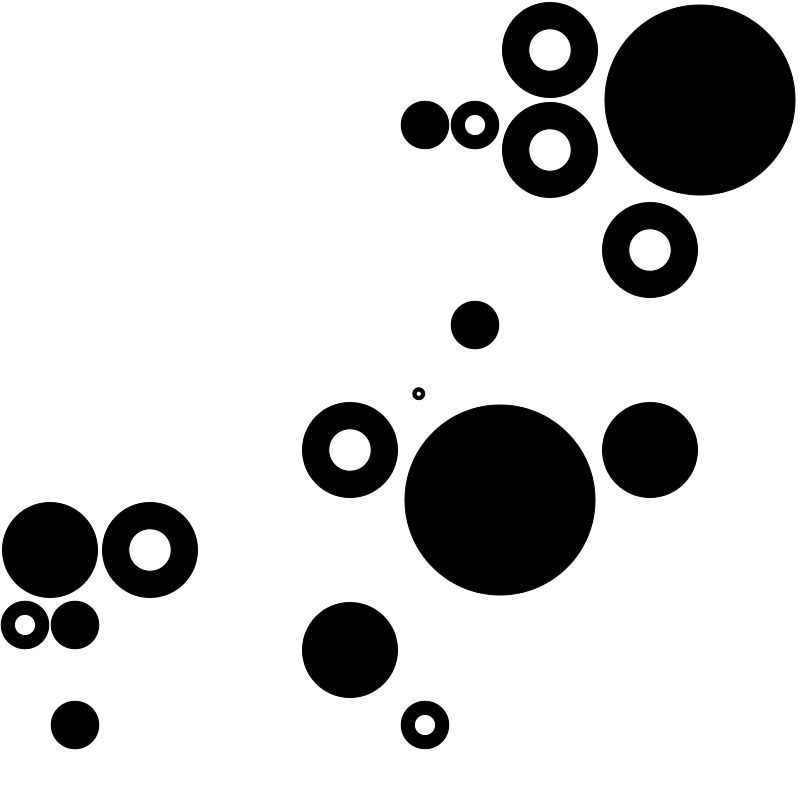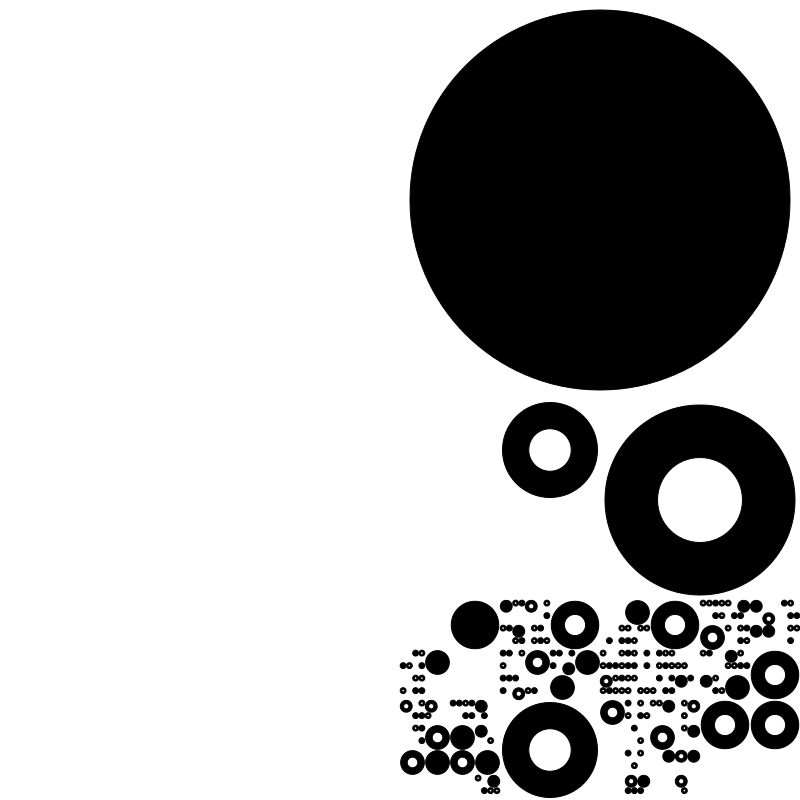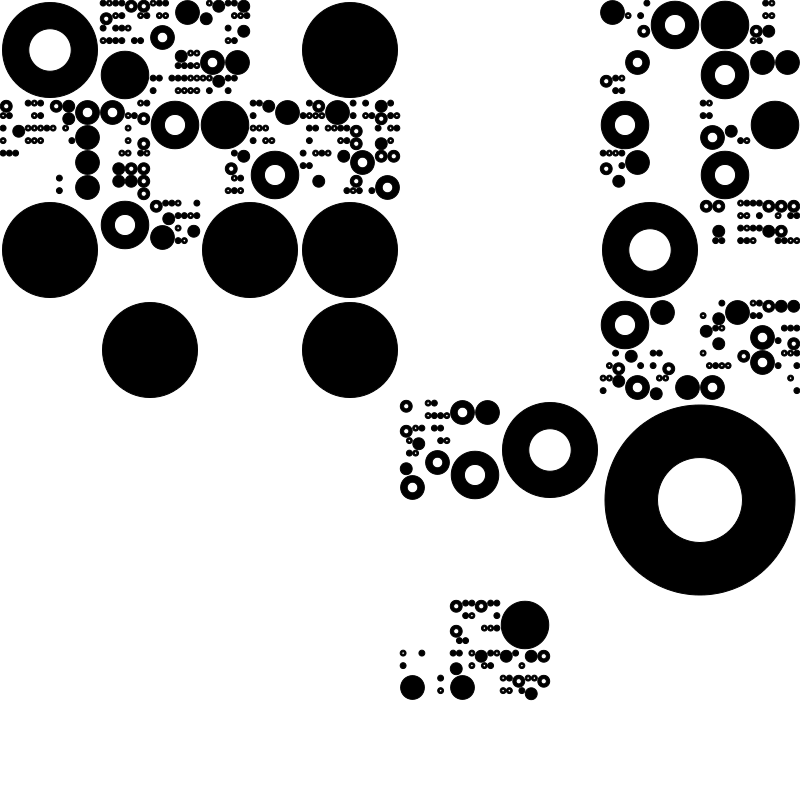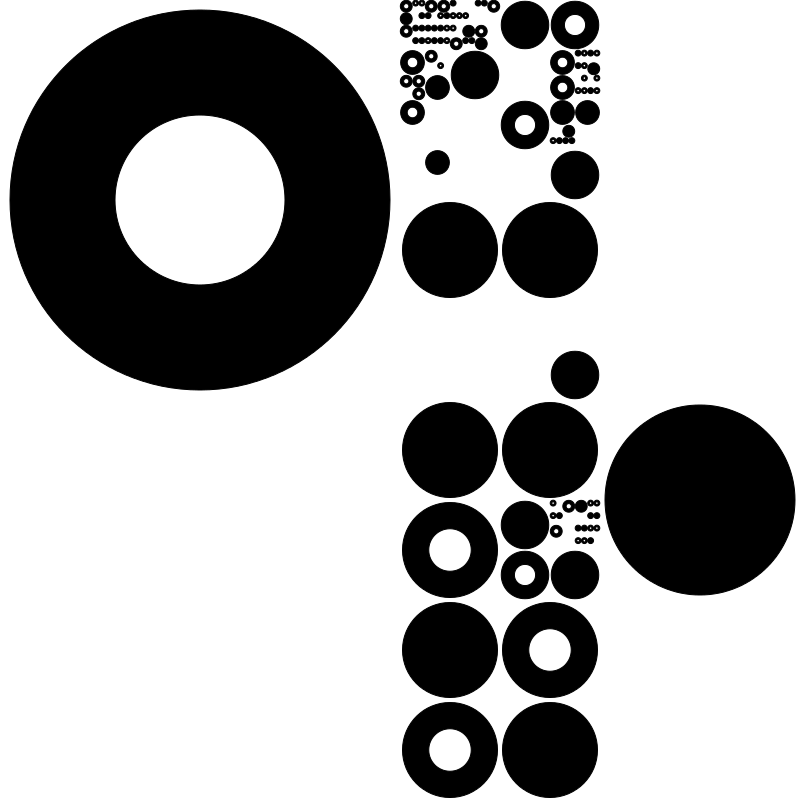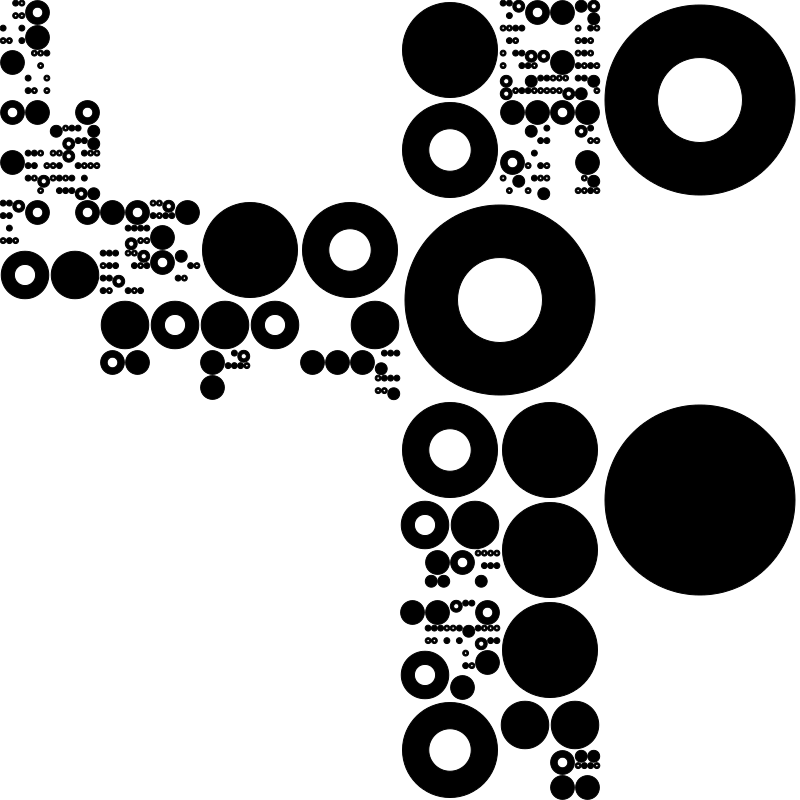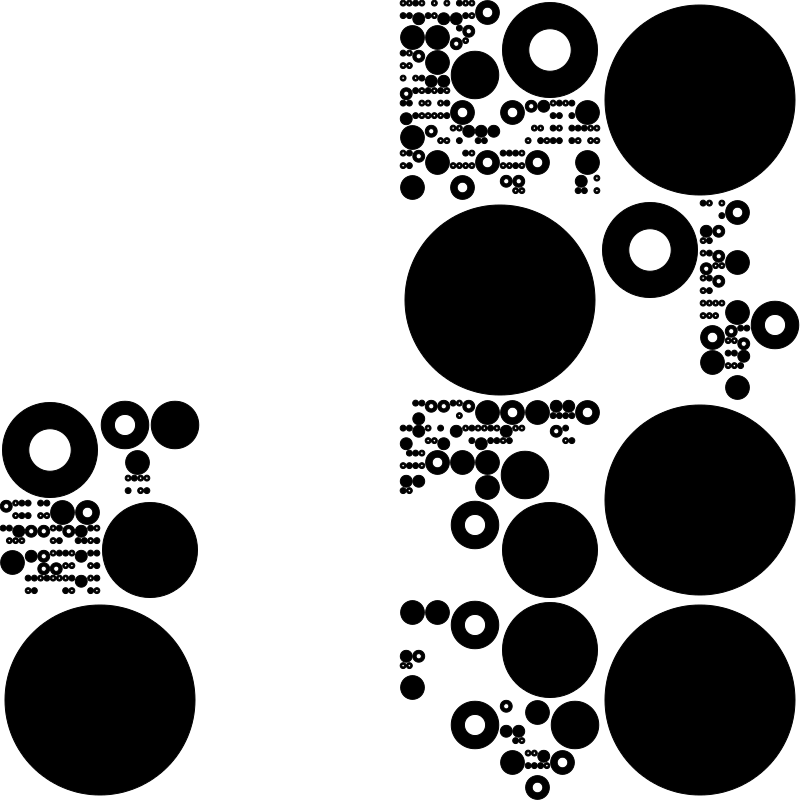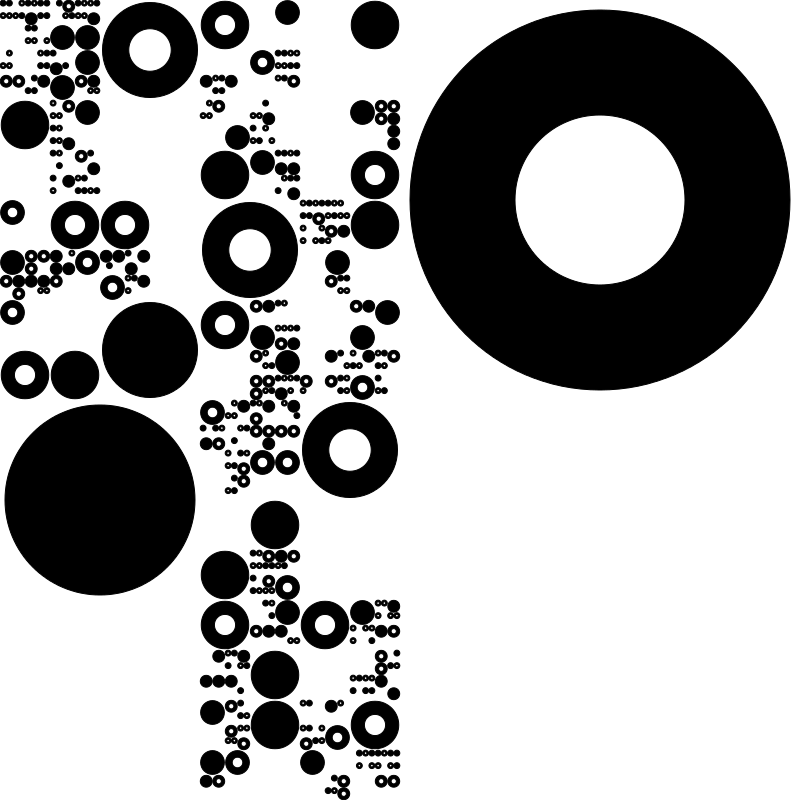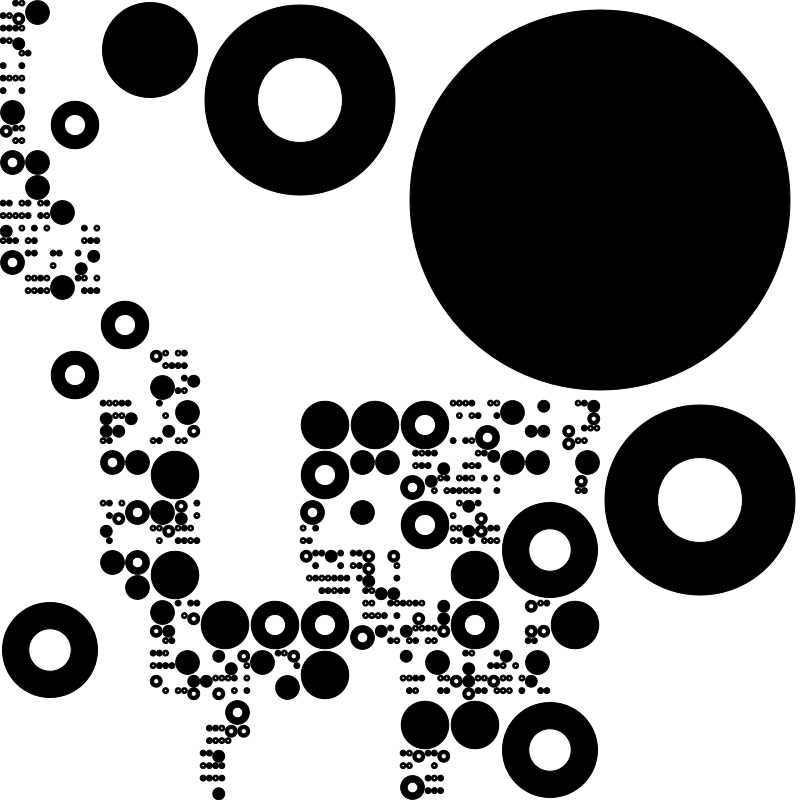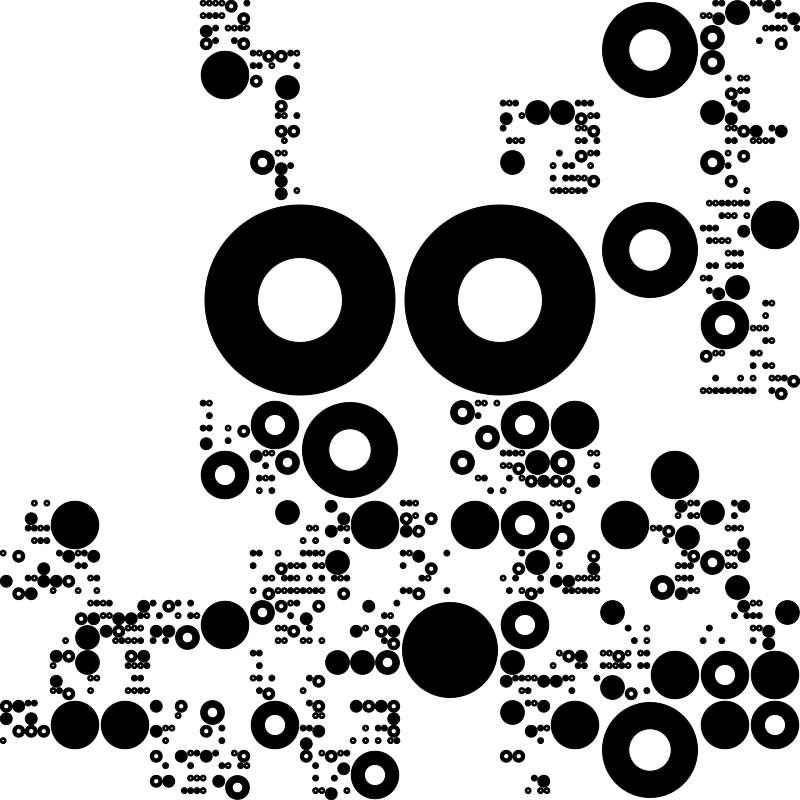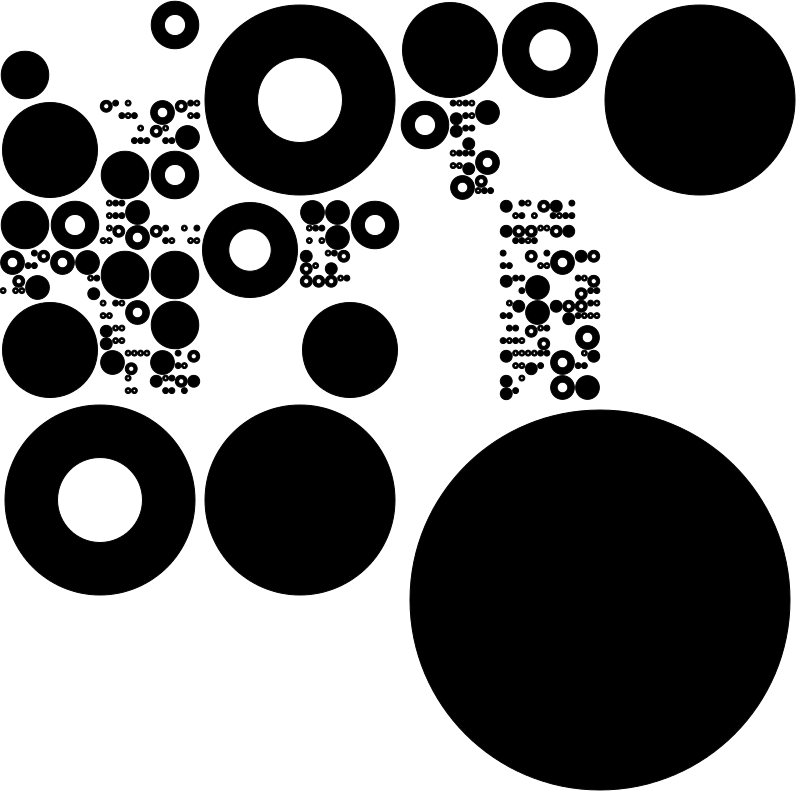It’s a simple recursive algorithm over a region of the sketch:

• Should we recurse?
• Yes, recurse:
• Is the region we’re looking at wider than tall? (The first time, this is the whole sketch.)
• Yes: split it into halves, vertically, and recurse into it.
• No: split it into halves, horizontally, and recurse into it.
• No, stop recursing:
• Should we draw a circle in this region?
• Yes: pick a circle type (solid or hollow) and draw it.

This generates a surprisingly wide variety of shapes, some very natural-looking.

There are three open-ended questions:

• Should we recurse?
• Should we draw a circle?
• What kind of circle?

If you decide to recurse every other time, and always draw a circle, and alternate the circles types, you get this image, which I made to help explain: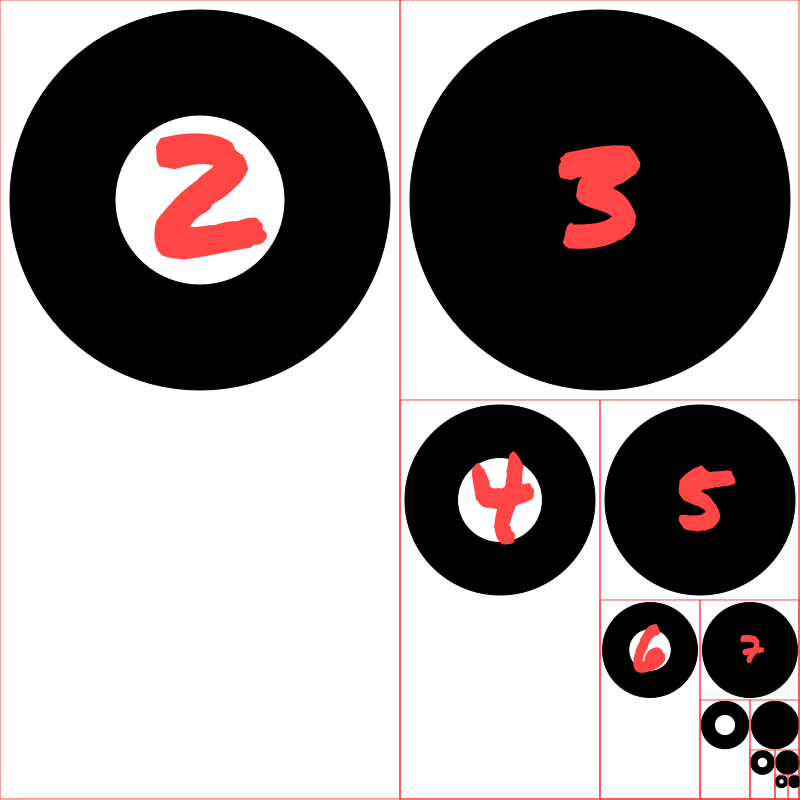If you let yourself pick the type of circle randomly, you’re left with two questions: whether to recurse, and whether to draw a circle. Answering those questions randomly, and controlling their probability, can generate whole families of images.

Here are 144 tiny fractal circle images. The probability of recursing decreases as we move from left to right, and the probability of drawing a circle increases as we move from top to bottom.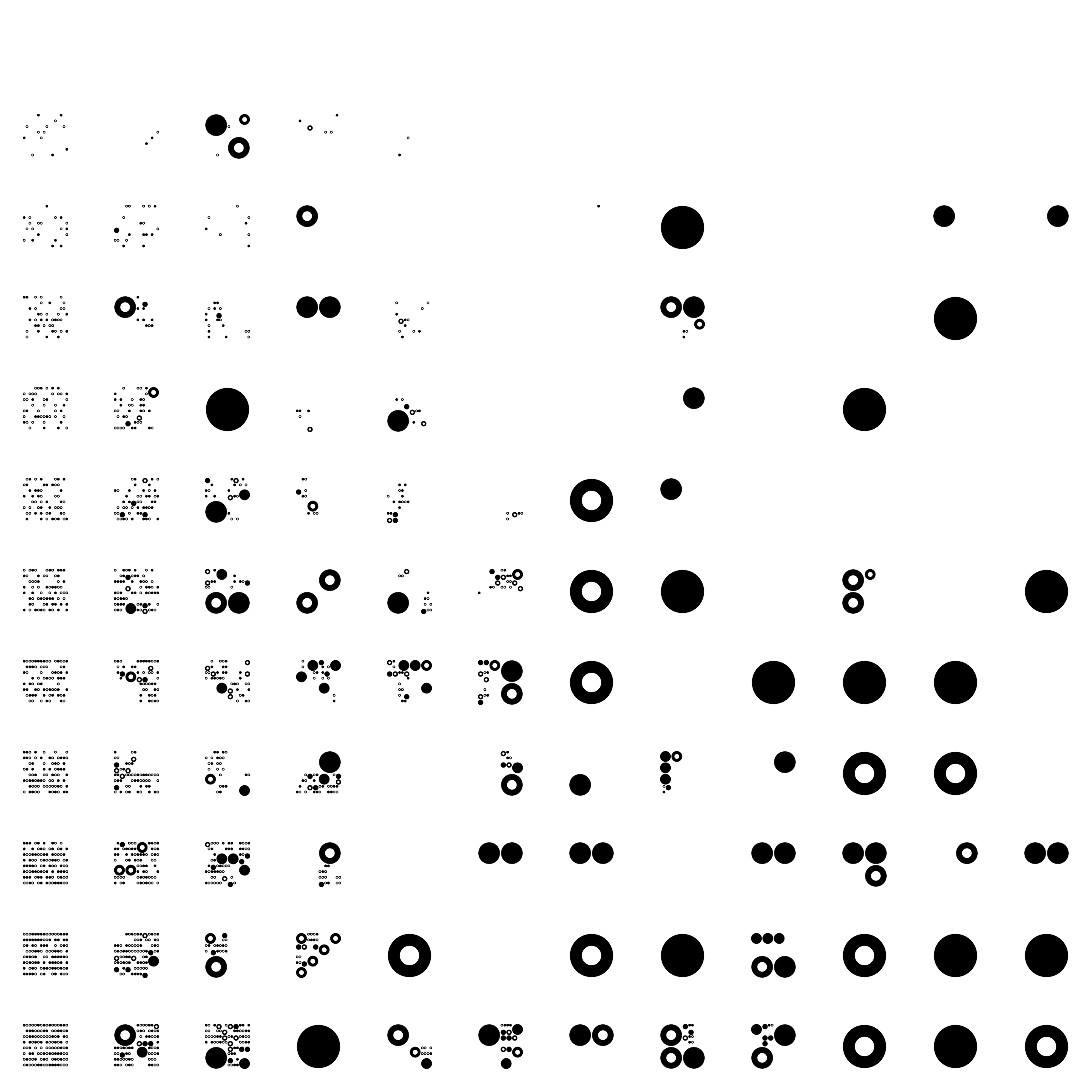Here’s a p5.js version of it, where the probabilty-to-recurse increases as the hour passes, and the probability-to-draw increases as the minute passes: